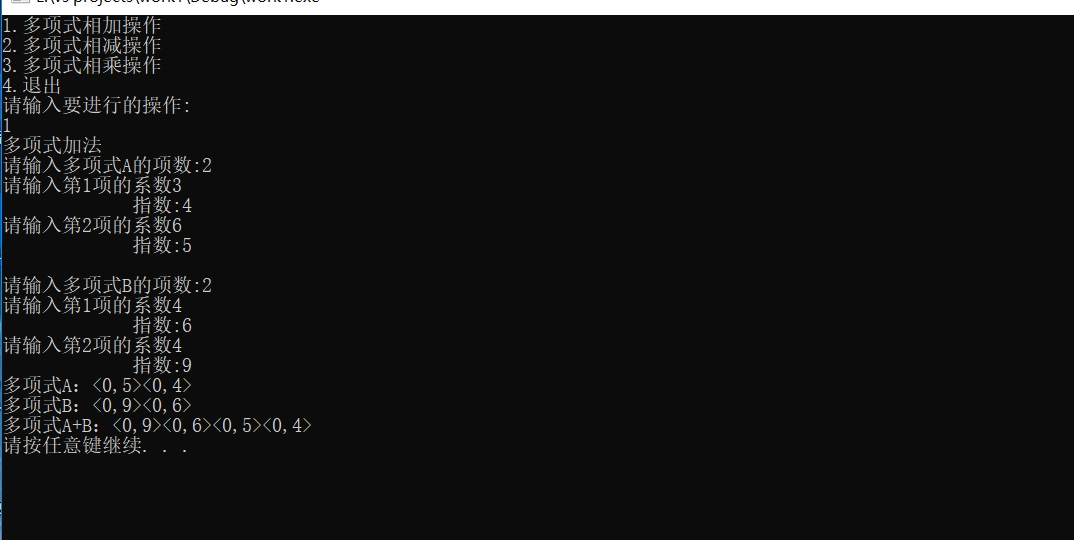``````#include<stdlib.h>
#include<malloc.h>
#include<math.h>
typedef struct ploy
{
float coef;   //系数部分
int expn;    //指数部分
struct ploy *next;
}ploy,*Ploy;    //结点类型，指针类型

void InsertPloyNode(Ploy p,Ploy q)    //插入链表算法
{
if(p->coef==0) delete p;
else{
Ploy q1,q2;
q1=q;
q2=q->next;
while(q2&&p->expn<q2->expn)
{
q1=q2;
q2=q2->next;
}
if(q2&&p->expn==q2->expn)
{
q2->coef+=p->coef;
delete p;
if(!q2->coef)
{
q1->next=q2->next;
delete q2;
}
}
else
{
p->next=q2;
q1->next=p;
}
}
}
{
int i;
Ploy p;
for(i=0;i<m;i++){
p=(Ploy)malloc(sizeof(struct ploy));
printf("请输入第%d项的系数",i+1);
scanf("%f",&p->coef);
printf("             指数:");
scanf("%d",&p->expn);
}

}
void FreePolyNode(Ploy p)   //释放多项式内存空间
{
Ploy p1;
while(p!=NULL)
{
p1=p;
p=p->next;
delete p1;
}
}
void PrintPoly(Ploy pf)  //输出多项式算法
{
Ploy qa=pf->next;
while (qa)
{
if(qa->coef!=1&&qa->coef!=-1)
{
printf("<%d",qa->coef);
if(qa->expn==1)
printf(",1>");
else if(qa->expn)
printf(",%d>",qa->expn);
}
else
{
if(qa->coef==1)
{
if(!qa->expn)
printf("<1,0>");
else if(qa->expn==1)
printf("<1,1>");
else printf("<1,%d>",qa->expn);
}
if(qa->coef==-1)
{
if(!qa->expn) printf("<-1,0>");
else if(qa->expn==1)
printf("<-1,1>");
else printf("-1,-%d>",qa->expn);
}
}
qa=qa->next;
}
printf("\n");
}
int SortPloy(Ploy a,Ploy b) //链表进行降序序排序算法
{
if(a&&b)
{
if(!b||a->expn>b->expn) return 1;
else if(!a||a->expn<b->expn) return -1;
else return 0;
}
else if(!a&&b) return -1;
else return 1;
}
{
Ploy qa=pa->next;
Ploy qb=pb->next;
q1=new ploy;
q1->next=NULL;
while(qa||qb)
{
sum=new ploy;
switch(SortPloy(qa,qb))
{
case 1:
{
sum->coef=qa->coef;
sum->expn=qa->expn;
qa=qa->next;
break;
}
case 0:
{
sum->coef=qa->coef+qb->coef;
sum->expn=qa->expn;
qa=qa->next;
qb=qb->next;
break;
}
case -1:
{
sum->coef=qb->coef;
sum->expn=qb->expn;
qb=qb->next;
break;
}
}
if(sum->coef!=0)
{
sum->next=q1->next;
q1->next=sum;
q1=sum;
}
else delete sum;
}

}
Ploy Subtract_Poly_Item( Ploy pa, Ploy pb)//多项式相减运算
{
Ploy pc=pb;
Ploy p=pb->next;
Ploy pp;
while(p)
{
p->coef*=-1;
p=p->next;
}
for(p=pc->next;p;p=p->next)
p->coef*=-1;
return pp;

}
Ploy Multiply_Poly_Item( Ploy pa,Ploy pb)//多项式相乘运算
{
Ploy p1,p2;//
Ploy qa=pa->next; //pa的后继结点
Ploy qb=pb->next; //pb的后继结点
p2=new ploy;
p2->next=NULL;
while(qa)       //项式的每一项都运算
{
qb=pb->next;
while(qb)
{
p1=new ploy;
p1->coef=qa->coef*qb->coef;
p1->expn=qa->expn+qb->expn;
InsertPloyNode(p1,p2);   //调用插入函数，合并同类项
qb=qb->next;
}
qa=qa->next;
}

return p2;//返回所得链表的头指针

}
Ploy PA,PB;
void input(){
int m,n;

printf("请输入多项式A的项数:");
scanf("%d",&m);
PA=CreatPloyNode(PA,m);
printf("\n");
printf("请输入多项式B的项数:");
scanf("%d",&n);
PB=CreatPloyNode(PB,n);}

void main()
{
int num;
printf("1.多项式相加操作 \n");
printf("2.多项式相减操作   \n");
printf("3.多项式相乘操作   \n");
printf("4.退出\n");
printf("请输入要进行的操作:");
printf("\n");
scanf("%d",&num);
switch(num)
{
case 1:
printf("多项式加法\n");
input();
printf("多项式A：");
PrintPoly(PA);
printf("多项式B：");
PrintPoly(PB);
printf("多项式A+B：");
break;
case 2:
printf("多项式减法\n");
input();
printf("多项式A：");
PrintPoly(PA);
printf("多项式B：");
PrintPoly(PB);
printf("多项式A-B：");
PrintPoly( Subtract_Poly_Item(PA,PB));
break;
case 3:
printf("多项式乘法\n");
input();
printf("多项式A：");
PrintPoly(PA);
printf("多项式B：");
PrintPoly(PB);
printf("多项式A*B：");
PrintPoly(Multiply_Poly_Item(PA,PB));
break;
case 4:
printf("您已退出此系统\n");
FreePolyNode(PA);
FreePolyNode(PB);
exit(0);
break;
default:
printf("输入错误\n");

}
system("pause");